# RS Aggarwal Solutions Class 10 Ex 19E

## RS Aggarwal Class 10 Ex 19E Chapter 19

The RS Aggarwal Class 10 solutions help students in clearing the concepts of the topic and practicing questions in order to prepare effectively for the exams. The solutions for Class 10 RS Aggarwal provide easy answers to the questions asked in the RS Aggarwal maths textbook. With the help of these solutions, scoring marks will become easier as it provides complete detailed explanations. The solutions are prepared by experienced subject experts in accordance to the CBSE syllabus.

These solutions will help you in your exam preparation and plan your study in a constructive way. Students can refer to these solutions whenever they get stuck or have any doubt while solving the questions. The RS Aggarwal Class 10 Solutions Chapter 19 Volume And Surface Areas Of Solids are highly effective from the exam point of view.

## Download PDF of RS Aggarwal Class 10 Solutions Chapter 19 – Volume And Surface Areas Of Solids Ex 19 E (19.5)

Question 1: The radii of the circular ends of a bucket of height 15 cm are 14 cm and r cm (r < 14). If the volume of the bucket is 5390 cm3, find the value of r.

Solution:

We have,

Height, h = 15 cm,

Radius of the upper end, R = 14 cm,

Radius of lower end = r,

As,

Volume of the bucket = 5390 cm3

$\Rightarrow$ $\frac{1}{3}\Pi h(R^{2}+r^{2}+Rr)$ = 5390

$\Rightarrow$ $\frac{1}{3}\times \frac{22}{7}\times 15\times (14^{2}+r^{2}+14r)$ = 5390

$\Rightarrow$ $\frac{110}{7}\times (196+r^{2}+14r)$ = 5390

$\Rightarrow$ 196 + r2 + 14r = $\frac{5390\times 7}{110}$

$\Rightarrow$ 196 + r2 + 14r = 343

$\Rightarrow$ r2 + 14r – 147 = 0

$\Rightarrow$ r2 + 21r – 7r – 147 = 0

$\Rightarrow$ r(r + 21) – 7(r + 21) = 0

$\Rightarrow$ (r + 21)(r – 7) = 0

$\Rightarrow$ r + 21 = 0 or r – 7 = 0

$\Rightarrow$ r = -21 or r = 7

As, r cannot be negative

Therefore,  r = 7 cm

So, the value of r is 7 cm.

Question 2: The radii of the circular ends of a solid frustum of a cone are 33 cm and 27 cm and its slant height is 10 cm. Find its total surface area. [Use $\Pi =3.14$]

Solution:

Greater radius = R = 33 cm

Smaller radius = r = 27 cm

Slant height = l = 10 cm

Surface area of the frustum

= $\Pi R^{2}+\Pi r^{2}+\Pi l(R+r)$

= $\Pi \left [ R^{2}+r^{2}+l(R+r) \right ]$

= $\Pi \left [ 33^{2}+27^{2}+10(33+27) \right ]$

= $\Pi \left [ 1089+729+10(60) \right ]$

= 2418 x 3.14

= 7592.52 cm2

Question 3: A tent is made in the form of a frustum of a cone surmounted by another cone. The diameters of the base and the top of the frustum are 20 m and 6 m respectively, and the height is 24 m. If the height of the tent is 28 m and the radius of the conical part is equal to the radius of the top of the frustum, find the quantity of canvas required. [Take $\Pi =\frac{22}{7}$]

Solution:

For the lower portion of the tent:

Diameter of the base = 20 m

Radius, R, of the base = 10 m

Diameter of the top end of the frustum = 6 m

Radius of the top end of the frustum = r = 3 m

Height of the frustum = h = 24 m

Slant height = l

= $\sqrt{h^{2}+(R-r)^{2}}$

= $\sqrt{24^{2}+(10-3)^{2}}$

= $\sqrt{576+49}$

= $\sqrt{625}$ = 25 m

For the conical part:

Radius of the cone’s base = r = 3 cm

Height of the cone = Total height – Height of the frustum = 28 – 24 = 4 m

Slant height, L, of the cone = $\sqrt{3^{2}+4^{2}}$ = $\sqrt{9+16}$ = $\sqrt{25}$ = 5 m

Total quantity of canvas = Curved surface area of the frustum + Curved surface area of the conical top

= $(\Pi l(R+r))+\Pi Lr$

= $\Pi (l(R+r)+Lr)$

= $\frac{22}{7}(25\times 13+5\times 3)$

= $\frac{22}{7}(325+15)$ = 1068.57 m2

Question 4: A tent consists of a frustum of a cone, surmounted by a cone. If the diameters of the upper and lower circular ends of the frustum be 14 m and 26 m respectively, the height of the frustum be 8 m and the slant height of the surmounted conical portion be 12 m, find the area of the canvas required to make the tent. (Assume that the radii of the upper circular end of the frustum and the base of the surmounted conical portion are equal.)

Solution:

For the frustum:

Upper diameter = 14 m

Upper Radius, r = 7 m

Lower Diameter = 26 m

Lower Radius, R = 13 m

Height of the frustum = h = 8 m

Slant height l = $\sqrt{h^{2}+(R-r)^{2}}$

= $\sqrt{8^{2}+(13-7)^{2}}$

= $\sqrt{64+36}$

= $\sqrt{100}$ = 10 m

For the conical part:

Radius of the base = r = 7 m

Slant height = L = 12 m

Total surface area of the tent = Curved area of frustum + Curved area of the cone

= $\Pi l(R+r)+\Pi rL$

= $\frac{22}{7}\times 10(13+7)+\frac{22}{7}\times 7\times 12$

= $\frac{22}{7}(200+84)$ = 892.57 m2

Question 5: The perimeters of the two circular ends of a frustum of a cone are 48 cm and 36 cm. If the height of the frustum is 11 cm, find its volume and curved surface area.

Solution:

We have,

Perimeter of upper end, C = 48 cm,

Perimeter of lower end, c = 36 cm and

Height, h = 11 cm

Let the radius of upper end be R and the radius of lower end be r.

As, C = 48 cm

$\Rightarrow$ $2\Pi r=48$

$\Rightarrow$ $R=\frac{48}{2\Pi }$

$\Rightarrow$ $R=\frac{24}{\Pi }cm$

Similarly, c = 36 cm

$\Rightarrow$ $r=\frac{36}{2\Pi }$

$\Rightarrow$ $r=\frac{18}{\Pi }cm$

And, l = $\sqrt{(R-r)^{2}+h^{2}}$

= $\sqrt{\left ( \frac{24}{\Pi }-\frac{18}{\Pi } \right )^{2}+11^{2}}$

= $\sqrt{\left ( \frac{6}{\Pi } \right )^{2}+11^{2}}$

= $\sqrt{\left ( \frac{6\times 7}{22} \right )^{2}+11^{2}}$

= $\sqrt{\left ( \frac{21}{11} \right )^{2}+11^{2}}$

= $\sqrt{\frac{441+121\times 121}{121}}$

= $\sqrt{\frac{441+14641}{121}}$

= $\frac{\sqrt{15082}}{11}cm$

Now,

Volume of the frustum = $\Rightarrow$ $\frac{1}{3}\Pi h(R^{2}+r^{2}+Rr)$

= $\frac{1}{3}\times \Pi \times 11\times \left [ \left ( \frac{24}{\Pi } \right )^{2}+\left ( \frac{18}{\Pi } \right )^{2}+\left ( \frac{24}{\Pi } \right )\times \left ( \frac{18}{\Pi } \right ) \right ]$

= $\frac{11\Pi }{3}\times \left [ \frac{576}{\Pi ^{2}}+\frac{324}{\Pi ^{2}}+\frac{432}{\Pi ^{2}} \right ]$

= $\frac{11\Pi }{3}\times \frac{1332}{\Pi ^{2}}$

= $\frac{11}{3}\times \frac{1332}{\Pi }$

= $\frac{11}{3}\times \frac{1332\times 7}{22}$

= 1554 cm3

Also,

Curved surface area of the frustum = $\Pi (R+r)l$

= $\frac{22}{7}\times \left ( \frac{22}{\Pi }+\frac{18}{\Pi } \right )\times \frac{\sqrt{15082}}{11}$

= $\frac{22}{7}\times \frac{42}{\Pi }\times \frac{\sqrt{15082}}{11}$

= $\frac{22}{7}\times \frac{42\times 7}{22}\times \frac{\sqrt{15082}}{11}$

$\approx 42\times 11.164436$

$\approx$ 468.91 cm2

Question 6: A solid cone of base radius 10 cm is cut into two parts through the midpoint of its height, by a plane parallel to its base. Find the ratio of the volumes of the two parts of the cone.

Solution: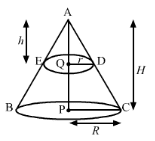We have,

Radius of solid cone, R = CP = 10 cm,

Let the height of the solid cone be, AP = H,

the radius of the smaller cone, QD = r and

the height of the smaller cone be, AQ = h.

Also, AQ = $\frac{AP}{2}$ i.e. h = $\frac{H}{2}$ or H = 2h – – – – – – – (i)

Now, in $\Delta$AQD and $\Delta$APC,

$\angle$QAD = $\angle$PAC (Common angle)

$\angle$AQD = $\angle$APC = 90o

So, by AA criteria

$\Delta$AQD $\sim$ $\Delta$APC

$\Rightarrow$ $\frac{AQ}{AP}=\frac{QD}{PC}$

$\Rightarrow$ $\frac{h}{H}=\frac{r}{R}$

$\Rightarrow$ $\frac{h}{2h}=\frac{r}{R}$ [Using (i)]

$\Rightarrow$ $\frac{1}{2}=\frac{r}{R}$

$\Rightarrow$ R = 2r – – – – – – – – – (ii)

As,

Volume of smaller cone = $\frac{1}{3}\Pi r^{2}h$

And,

Volume of solid cone = $\frac{1}{3}\Pi R^{2}H$

= $\frac{1}{3}\Pi (2r)^{2}\times (2h)$ [Using (i) and (ii)]

= $\frac{8}{3}\Pi r^{2}h$

So,

Volume of frustum = Volume of solid cone – Volume of smaller cone

= $\frac{8}{3}\Pi r^{2}h$$\frac{1}{3}\Pi r^{2}h$

= $\frac{7}{3}\Pi r^{2}h$

Now, the ratio of the volumes of the two parts = $\frac{Volume\, of\, the\, smaller\, cone}{Volume\, of\, the\, fructum}$

= $\frac{\left ( \frac{1}{3}\Pi r^{2}h \right )}{\left ( \frac{7}{3}\Pi r^{2}h \right )}$

= $\frac{1}{7}$

= 1 : 7

So, the ratio of the volume of the two parts of the cone is 1 : 7.

Question 7: The height of a right circular cone is 20 cm. A small cone is cut off at the top by a plane parallel to the base. If its volume is $\frac{1}{8}$ of the volume of the given cone, at what height above the base is the section made?

Solution:We have,

Height of the given cone, H = 20 cm

Let the radius of the given code be R,

the height of the smaller cone be h and

the radius of the smaller cone be r.

Now, in $\Delta$AQD and $\Delta$APC,

$\angle$QAD = $\angle$PAC (Common angle)

$\angle$AQD = $\angle$APC = 90o

So, by AA criteria

$\Delta$AQD $\sim$ $\Delta$APC

$\Rightarrow$ $\frac{AQ}{AP}=\frac{QD}{PC}$

$\Rightarrow$ $\frac{h}{H}=\frac{r}{R}$ – – – – – – – – (i)

Volume of smaller cone = $\frac{1}{8}$ x Volume of the given cone

$\Rightarrow$ $\frac{Volume\, of\, smaller\, cone}{Volume\, of\, the\, given\, cone}=\frac{1}{8}$

$\Rightarrow$ $\frac{\left ( \frac{1}{3}\Pi r^{2}h \right )}{\left ( \frac{1}{3}\Pi R^{2}H \right )}=\frac{1}{8}$

$\Rightarrow$ $\left ( \frac{r}{R} \right )^{2}\times \left ( \frac{h}{H} \right )=\frac{1}{8}$

$\Rightarrow$ $\left ( \frac{h}{H} \right )^{2}\times \left ( \frac{h}{H} \right )=\frac{1}{8}$ [Using (i)]

$\Rightarrow$ $\left ( \frac{h}{H} \right )^{3}=\frac{1}{8}$

$\Rightarrow$ $\frac{h}{H}=\sqrt{\frac{1}{8}}$

$\Rightarrow$ $\frac{h}{20}=\frac{1}{2}$

$\Rightarrow$ h = $\frac{20}{2}$

$\Rightarrow$ h = 10 cm

Therefore, PQ = H – h = 20 – 10 = 10 cm

So, the section is made at the height of 10 cm above the base.

Question 8: A solid metallic right circular cone 20 cm high and whose vertical angle is 60o, is cut into two parts at the middle of its height by a plane parallel to its base. If the frustum so obtained be drawn into a wire of diameter $\frac{1}{12}$ cm, find the length of the wire.

Solution: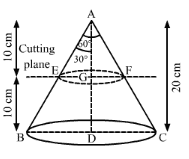We have,

Height of the solid metallic cone, H = 20 cm,

Height of the fructum, h = $\frac{20}{2}$ = 10 cm and

Radius of the wire = $\frac{1}{24}cm$

Let the length of the wire be l, EG = r and BD = R.

In $\Delta$AEG,

tan 30o = $\frac{EC}{AG}$

$\Rightarrow$ $\frac{1}{\sqrt{3}}=\frac{r}{H-h}$

$\Rightarrow$ $\frac{1}{\sqrt{3}}=\frac{r}{20-10}$

$\Rightarrow$ r = $\frac{10}{\sqrt{3}}cm$

Also, in $\Delta$ABD,

tan 30o = $\frac{BD}{AD}$

$\Rightarrow$ $\frac{1}{\sqrt{3}}=\frac{R}{H}$

$\Rightarrow$ $\frac{1}{\sqrt{3}}=\frac{R}{20}$

$\Rightarrow$ R = $\frac{20}{\sqrt{3}}cm$

Now,

Volume of the wire = Volume of the frustum

$\Rightarrow$ $\Pi \left ( \frac{1}{24} \right )^{2}l=\frac{1}{3}\Pi h(R^{2}+r^{2}+Rr)$

$\Rightarrow$ $\frac{l}{576}=\frac{1}{3}\times 10\times \left [ \left ( \frac{20}{\sqrt{3}} \right )^{2}+\left ( \frac{10}{\sqrt{3}} \right )^{2}+\left ( \frac{20}{\sqrt{3}} \right )\left ( \frac{10}{\sqrt{3}} \right ) \right ]$

$\Rightarrow$ $l=\frac{576}{3}\times 10\times \left [ \frac{400}{3}+\frac{100}{3}+\frac{200}{3} \right ]$

$\Rightarrow$ $l=\frac{576}{3}\times 10\times \frac{700}{3}$

$\Rightarrow$ l = 448000 cm

Thererfore, l = 4480 m

So, the length of the wire is 4480 m.

Question 9: A fez, the cap used by the Turks, is shaped like the frustum of a cone. If its radius on the open side is 10 cm, the radius at the upper base is 4 cm and its slant height is 15 cm, find the area of material used for making it. [Use $\Pi =\frac{22}{7}$]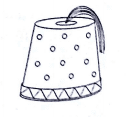Solution: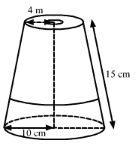We have,

Radius of open side, R = 10 cm,

Radius of upper base, r = 4 cm and

Slant height, l = 15 cm

Now,

The area of material used = $\Pi (R+r)l+\Pi r^{2}$

= $\frac{22}{7}\times (10+4)\times 15+\frac{22}{7}\times 4\times 4$

= $\frac{22}{7}\times 14\times 15+\frac{22}{7}\times 4\times 4$

= $\frac{22}{7}\times (14\times 15+4\times 4)$

= $\frac{22}{7}\times (210+16)$

= $\frac{22}{7}\times (226)$

= $\frac{4972}{7}cm^{2}$

$\approx 710.28cm^{2}$

So, the area of material used for making the fez is 710.28 cm2.

Question 10: An oil funnel made of tin sheet consists of a 10 cm long cylindrical portion attached to a frustum of a cone. If the total height is 22 cm, the diameter of the cylindrical portion is 8 cm and the diameter of the top of the funnel is 18 cm, find the area of the tin sheet required to make the funnel.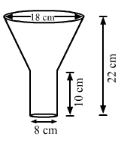Solution:

We have,

Height of the cylindrical portion, h = 10 cm,

Height of the frustum of cone portion, H = 22 – 10 = 12 cm,

Radius of the cylindrical portion = Radius of smaller end of frustum portion, r = $\frac{8}{2}$ = 4 cm and

Radius of larger end of frustum, R = $\frac{18}{2}$ = 9 cm

Also, the slant height of the frustum, l = $\sqrt{(R-r)^{2}+H^{2}}$

= $\sqrt{(9-4)^{2}+12^{2}}$

= $\sqrt{5^{2}+12^{2}}$

= $\sqrt{25+144}$

= $\sqrt{169}$

= 13 cm

Now,

The area of the tin sheet required = CSA of frustum of cone + CSA of cylinder

= $\Pi (R+r)l+2\Pi rh$

= $\frac{22}{7}\times (9+4)\times 13+2\times \frac{22}{7}\times 4\times 10$

= $\frac{22}{7}\times 13\times 13+\frac{22}{7}\times 80$

= $\frac{22}{7}\times (169+80)$

= $\frac{22}{7}\times 249$$\approx 782.57cm^{2}$

So, the area of the tin sheet required to make the funnel is 782.57 cm2.